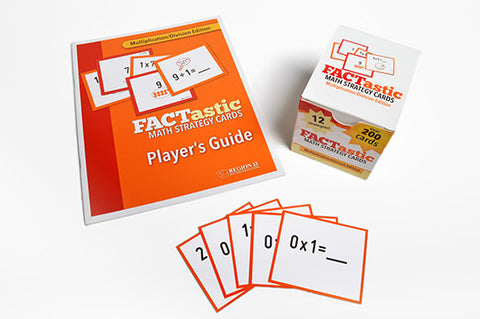## FACTastic Math Strategy System: Multiplication/Division (Set of Cards and Spiral-Bound Teacher's Guide)

• \$ 4500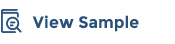FACTastic Math Strategy System: Multiplication/Division makes the basic instruction of multiplication and division for elementary-aged students more fun, while increasing true knowledge and fluency.

Our FACTastic Math Strategy System is a comprehensive program for teaching basic math facts through strategy-based instruction to build the flexible thinking and solid mathematical foundation that are critical for their success. The system includes:

• Model lessons that suggest how to teach each strategy through hands-on use of physical objects and visuals.
• Strategies and cards based on research that shows how concrete and visual representations can build students’ subject matter fluency.
• The FACTastic Teacher's Guide to walk teachers through the teaching of each strategy by emphasizing key points.
• Examples of think-aloud techniques in the Teacher's Guide.

##### Includes:

FACTastic Math Strategy System was produced and is maintained in collaboration with Region 13's Special Education team and experts from the field.

We Also Recommend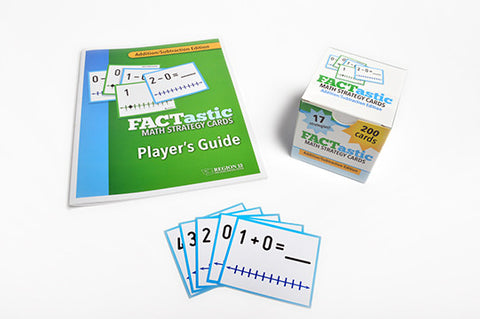FACTastic Math Strategy System: Addition/Subtraction (Set of Cards and Spiral-Bound Teacher's Guide)

\$ 45.00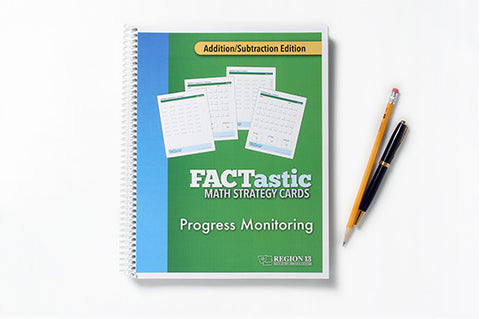FACTastic Math Progress Monitoring: Addition/Subtraction (Spiral-Bound)

\$ 20.00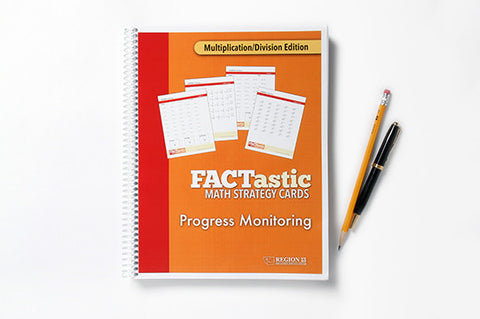FACTastic Math Progress Monitoring: Multiplication/Division (Spiral-Bound)

\$ 20.00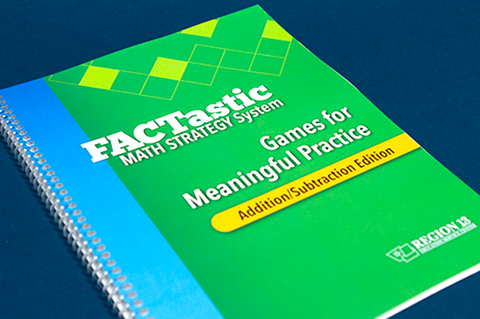FACTastic Math Strategy System Games for Meaningful Practice: Addition/Subtraction (Spiral-Bound)

\$ 30.00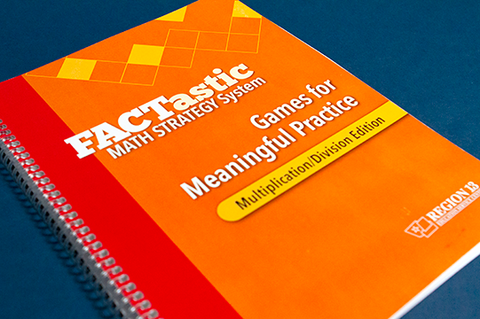FACTastic Math Strategy System Games for Meaningful Practice: Multiplication/Division (Spiral-Bound)

\$ 30.00
x